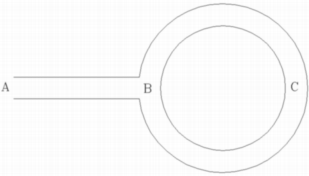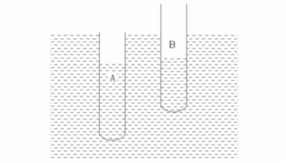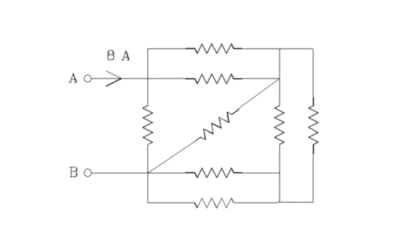PHYSICS

 Time: ONE Hour Max. Marks: 60
• Answers must be written either in English or the medium of instruction of the candidate in high school.
• Answer all the questions in the booklets provided for the purpose.
• There will be no negative marking.
• The relevant working in arriving at an answer has to be shown wherever required.
• Use of calculators or graph papers is not permitted.
• All questions carry equal marks
 A person leaves A at 6 AM, walks from A to B with a uniform speed of 4 kmph, from B to C at 6 kmph , C to B at 3 kmph and then back to A at 4 kmph. If the time interval between the moments he is at B is 90 minutes and he was at C at 7 AM, Find the time when he is returns to A.1. Two stones are located at the same height above the ground at a horizontal distance of 20 m. One of them is projected vertically upward at 20 m/s and the other dropped from rest at the same moment. Find the distance between them one second later. (Assume acceleration due to gravity is 10 ms–2 )

2. A small mass of 2 kg is tied to the end of a string attached to a fixed point. The mass is drawn aside such that the string is horizontal and is released. When it swings along a circular arc through an angle of 30o it has kinetic energy of 8 J. Find its kinetic energy when the string becomes vertical.

3. A car is taking a turn of radius 20 m at a speed of 36 kmph. Find the ratio of resultant force on the car to its weight.

4. A car starting from rest goes round a bend with its speed increasing linearly with time. If 2 s after start its centripetal acceleration is 4 m/s2 find its centripetal acceleration a second later.

5. A particle is going round a circular path making one revolution in 4 s. Consider any diameter of the circle and project the position of the particle going round the circle on to diameter. Times taken to move between two points on the circle and the time taken to move between the corresponding projections on the diameter are obviously the same. Find the time taken by the projection to move between the extreme of the diameter and a point midway between the center and the extreme.

6. A particle starting from rest from O accelerates uniformly along a straight line and crosses two points A and B which are 5 m apart in 2 s. If velocity at B is 2 m/s more than the velocity at A, find the distance OA.

 A thin test tube of negligible weight is floating in water ( density of water 1gm/cm3) with some liquid A filling part of its length to keep it upright. When the test tube is three fourth’s full it is just about to submerge as shown . When another liquid B fills half the length of the tube, one fourth of the length of the test tube is seen above water. Find the density of the two liquids1. A point object is located on the principal axis of a lens at a distance of 25 cm from it. When the object is moved 5 cm away from the lens the image moves by 40 cm. Find the focal length of the lens

2. Two wires made of same material have their thicknesses in the ratio 1:2 and have equal lengths. When connected in parallel, their equivalent resistance is R. Find the equivalent resistance when they are connected in series.

3. A magnet is arranged parallel to an external uniform magnetic field of induction Bo with its north pole pointing in the direction of the field. At a point on the equatorial line of the magnet the magnetic induction is zero. Find the induction at double this distance on the axial line of the magnet.

 Find the current in the diagonal resistance. All resistances are equal.1. A straight conductor carries a current vertically upward. A charged particle moving horizontally towards the wire is at an instant located to the east of the wire. Find the direction of the magnetic field at the position of the charged particle and the direction of the force on it.

2. A longitudinal wave of frequency 1000 Hz is travelling in a medium at 350 m/s. At a certain point in the medium there is a compression at a certain moment. How many milliseconds later would the next rarefaction form at this place. How far is the earlier compression at this time.

3. Fission of one uranium nucleus evolves 200 MeV of energy. Find the number of nucleii of uranium that should undergo fission to evolve energy required to power a 40 W lamp for 25 hrs. How many micrograms of mass has disappeared in the process.